Next: Normal Mode Wavefunctions Up: RESULTS: SQUARE LATTICE Previous: Size and Vortex

## Scaling With System Size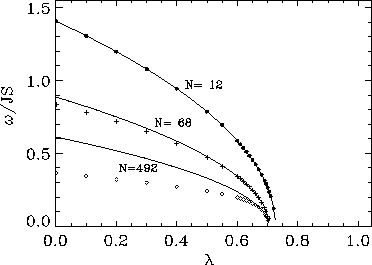Figure 5:   Asymptotic least square fits (solid curves) of the frequency of the soft mode (data points) for square lattice circular systems with 12, 68, and 492 spins, using the functional form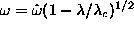. Only the data with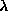very close to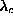were used to obtain the fitted curves. This functional form fits well over the full range ofonly for small systems (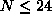).

For fixed boundary conditions, the soft mode for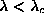has been fit to the following functional form, as suggested through the simple Ansatz by Wysin ;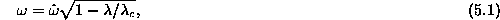where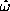andare the fitting parameters. Generally, if all the data where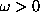are used in the fit, this functional form produces an accurate fit only for the smaller systems, up to about 24 spins. More generally, we can use only a limited number of the data points nearest to the zero frequency point, and apply this form there to estimate. Some typical asymptotic fits are shown in Fig. 5, for systems with 12, 68, and 492 spins. The values ofdetermined this way converge to a limit near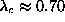for the infinite sized system. The frequency of the soft mode at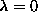,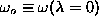, gives an indication of the overall frequency scale for this mode, and is shown in Fig. 6, versus system size (numbers not obtained from any fitting). The result is compared with an asymptotic fit to the function,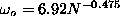. This is close to a linear dependence on inverse system length.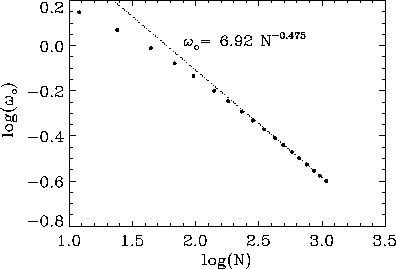Figure 6:   Size dependence of the soft mode for square lattice circular systems with N sites, using fixed boundary conditions. The frequency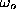of the soft mode atis shown on a log-log plot, and compared with an asymptotic fit to the function,. This is close to a linear dependence on inverse system diameter.Next: Normal Mode Wavefunctions Up: RESULTS: SQUARE LATTICE Previous: Size and Vortex

Gary M Wysin
Mon Sep 11 12:02:10 CDT 1995